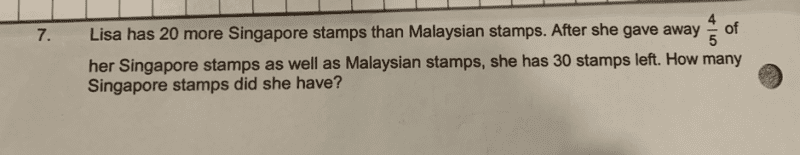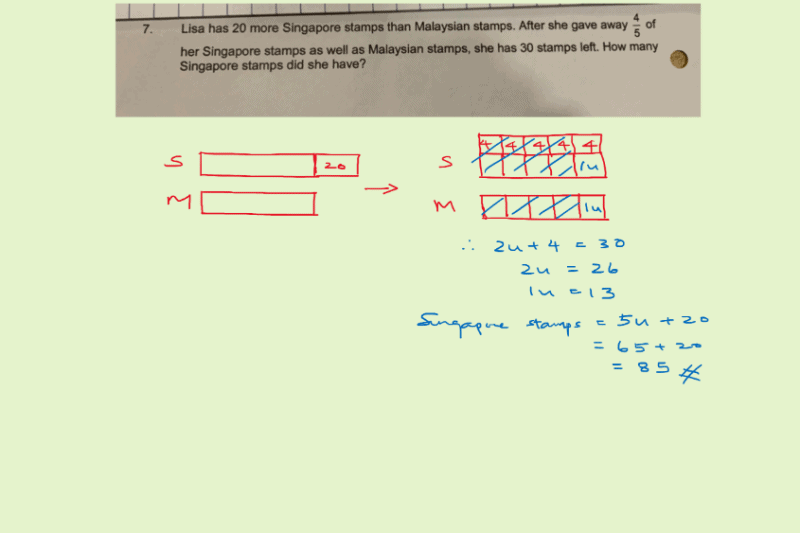# QuestionThank you so much!

7.

Let S be the number of Singapore stamps Lisa has at first.
Let M be the number of Malaysian stamps Lisa has at first.

S – M = 20 … (1)

1/5 ( S + M) = 30
S + M = 150 … (2)

(1) + (2): 2S = 170
=> S = 85#